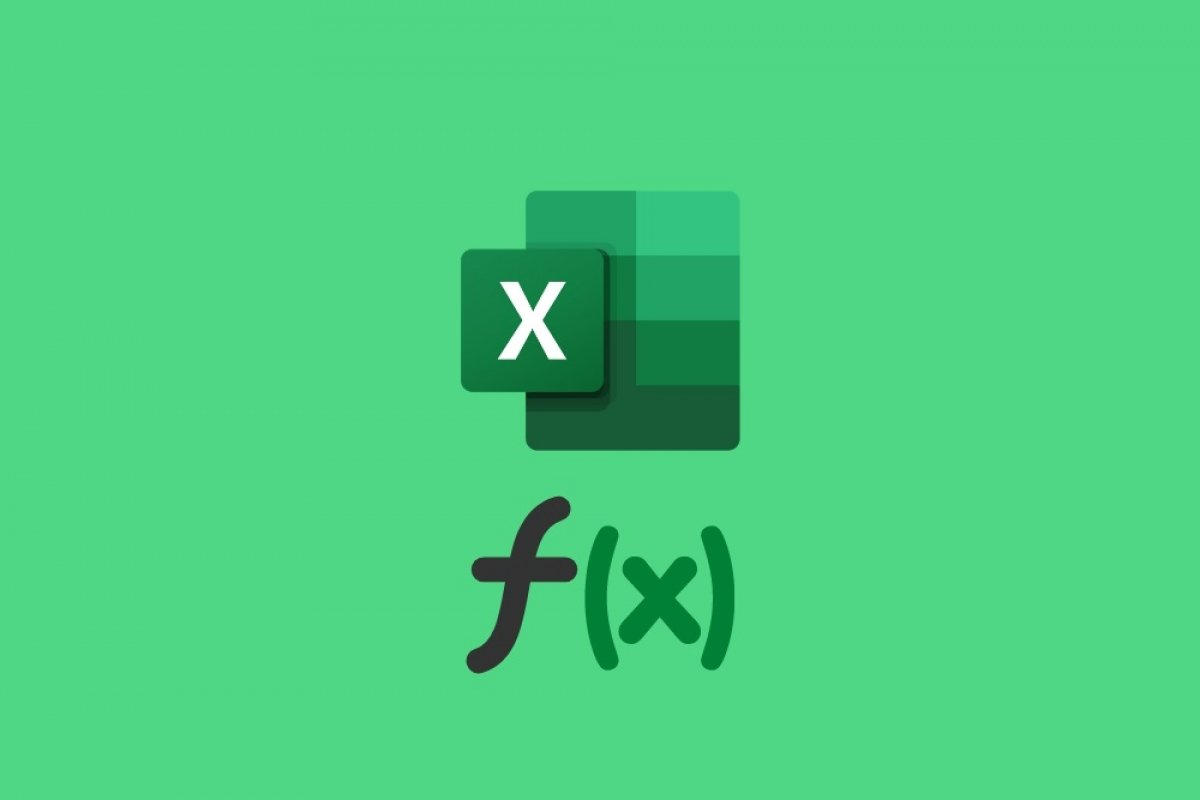A formula in Excel is the written instruction that tells the application what type of calculation to perform. To use them, it is necessary to type it in the formula bar, after having selected a cell. It is also possible to enter it directly into the cell.

If you want to use the formulas in Excel, you need to inform the application in a specific way. Therefore, when you are about to write a formula, first of all, enter the = (equal) sign. If this character is not used, Excel will interpret that you are adding plain text or a custom number. It is important to know that the formulas are not visible to the naked eye. Rather, in those cells where the formula has been used, the result is displayed. To view or edit the formula, double-click on the cell or use the upper formula bar.

Each formula has its own syntax. This means that the user must enter the data in a specific order and with specific criteria. For example, if you want to sum a range of cells, you should use the SUM formula as follows:

=SUMA(A1:B3)

To indicate the interval, in this case, the symbol : (colon) must be used. If you use any other, Excel will not understand what you are referring to.

A formula can be simple and perform simple operations (=A1+A2 or =A1-A2). It can also be complex and with variables (=SI(condition, result if met, result if not met). In any case, the key to getting the most out of the formulas is their continued use. As you learn more formulas, you will be able to get more out of Excel and create more complete spreadsheets. Remember that, if you are not clear, you can find an explanation of each formula, as well as the necessary syntax, in the Excel help section.